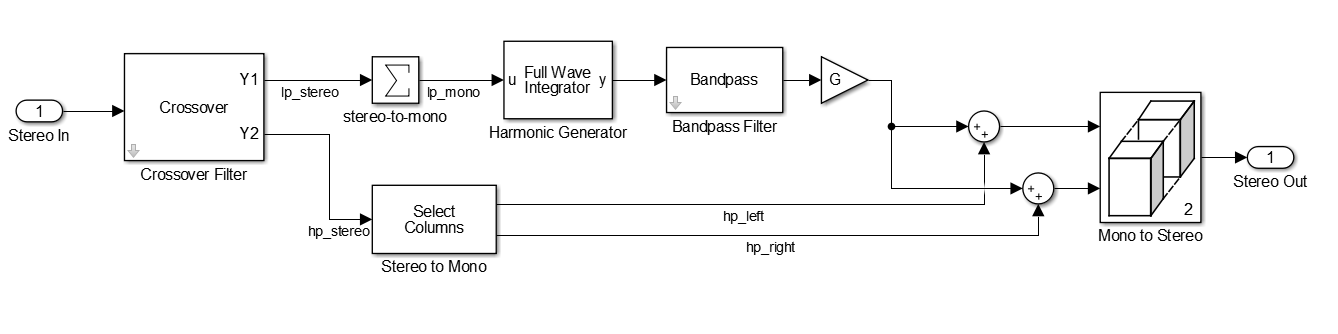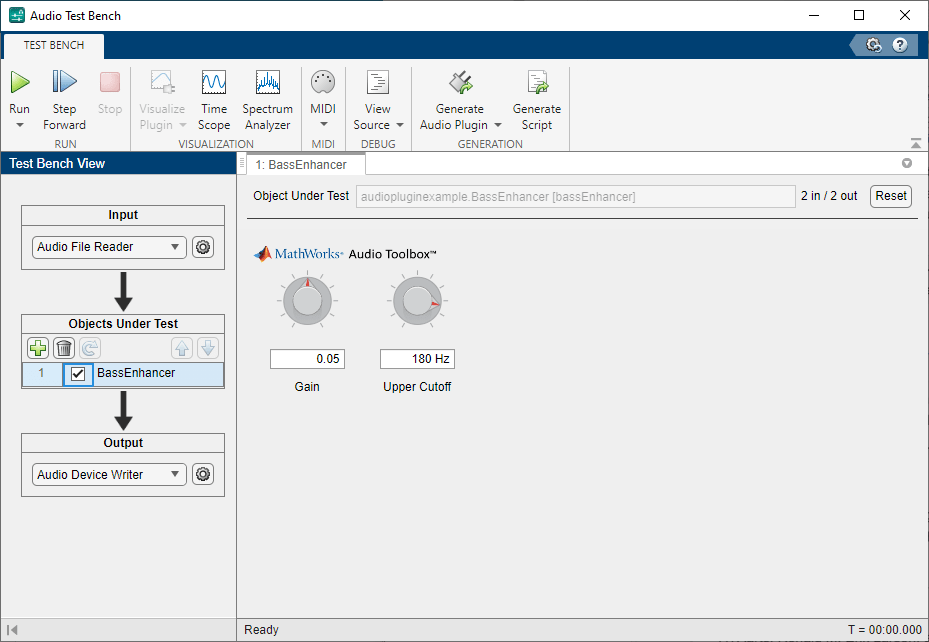# Psychoacoustic Bass Enhancement for Band-Limited Signals

This example shows an audio plugin designed to enhance the perceived sound level in the lower part of the audible spectrum.

### Introduction

Small loudspeakers typically have a poor low frequency response, which can have a negative impact on overall sound quality. This example implements psychoacoustic bass enhancement to improve sound quality of audio played on small loudspeakers.

The example is based on the algorithm in . A non-linear device shifts the low-frequency range of the signal to a high-frequency range through the generation of harmonics. The pitch of the original signal is preserved due to the "virtual pitch" psychoacoustic phenomenon.

The algorithm is implemented using an audio plugin object.

### Algorithm

The figure below illustrates the algorithm used in .1. The input stereo signal is split into lowpass and highpass components using a crossover filter. The filter's crossover frequency is equal to the speaker's cutoff frequency (set to 60 Hz in this example).

2. The highpass component, $h{p}_{stereo}$, is split into left and right channels: $h{p}_{left}$ and $h{p}_{right}$, respectively.

3. The lowpass component, $l{p}_{stereo}$, is converted to mono, $l{p}_{mono}$, by adding the left and right channels element by element.

4. $l{p}_{mono}$ is passed through a full wave integrator. The full wave integrator shifts $l{p}_{mono}$ to higher harmonics.• $u\left[n\right]$ is the input signal, $l{p}_{mono}$

• $y\left[n\right]$ is the output signal

• $n$ is the time index

5. $y\left[n\right]$ is passed through a bandpass filter with lower cutoff frequency set to the speaker's cutoff frequency. The bandpass's upper cutoff frequency may be adjusted to fine-tune output sound quality.

6. ${y}_{BP}\left[n\right]$, the bandpass filtered signal, passes through tunable gain, $G$.

7. ${y}_{G}$ is added to the left and right highpass channels.

8. The left and right channels are concatenated into a single matrix and output.

Although the resulting output stereo signal does not contain low-frequency elements, the input's bass pitch is preserved thanks to the generated harmonics.

### Bass Enhancer Audio plugin

audiopluginexample.BassEnhancer is an audio plugin object that implements the psychoacoustic bass enhancement algorithm. The plugin parameters are the upper cutoff frequency of the bandpass filter, and the gain applied at the output of the bandpass filter (G in the diagram above). You can incorporate the object into a MATLAB simulation, or use it to generate an audio plugin using `generateAudioPlugin`.

You can open a test bench for `audiopluginexample.BassEnhancer` using Audio Test Bench. The test bench provides a graphical user interface to help you test your audio plugin in MATLAB. You can tune the plugin parameters as the test bench is executing. You can also open a `dsp.TimeScope` and a `dsp.SpectrumAnalyzer` to view and compare the input and output signals in the time and frequency domains, respectively.

```bassEnhancer = audiopluginexample.BassEnhancer; audioTestBench(bassEnhancer)```You can also use `audiopluginexample.BassEnhancer` in MATLAB just as you would use any other MATLAB object. You can use `configureMIDI` to enable tuning the object using a MIDI device. This is particularly useful if the object is part of a streaming MATLAB simulation where the command window is not free.

`HelperBassEnhancerSim` is a simple function that may be used to perform bass enhancement as part of a larger MATLAB simulation. The function instantiates an `audiopluginexample.BassEnhancer` plugin, and uses the `setSampleRate` method to set its sampling rate to the input argument `Fs`. The plugin's parameters are tuned by setting their values to the input arguments `Fcutoff` and `G`, respectively. Note that it is also possible to generate a MEX-file from this function using the `codegen` command. Performance is improved in this mode without compromising the ability to tune parameters.

### References

 Aarts, Ronald M, Erik Larsen, and Daniel Schobben. “Improving Perceived Bass and Reconstruction of High Frequencies for Band Limited Signals.” Proceedings 1st IEEE Benelux Workshop on Model Based Coding of Audio (MPCA-2002) , November 15, 2002, 59–71.

##### Support